# 机器学习：对数几率回归-1

(在苹果系统下，如果文章中的图片不能正常显示，请升级Safari浏览器到最新版本，或者使用Chrome、Firefox浏览器打开。)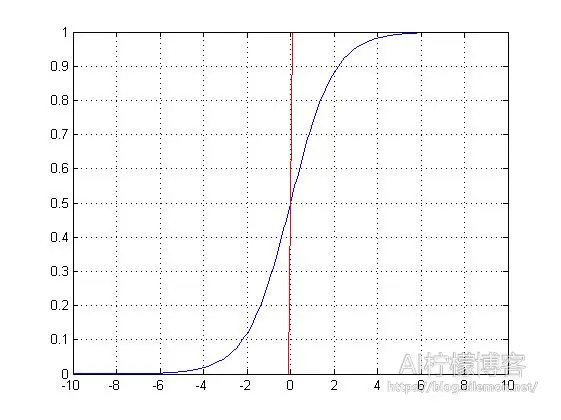Sigmoid函数（蓝）与单位阶跃函数（红）

我们刚才说，对数几率回归是用来实现分类任务的，包括基于此的感知机（Perceptron）和神经网络模型（Neural Network model），都是用来进行分类的。我们给定一个输入值，如果y是样本x作为正例的可能性，那么1 – y就是其反例的可能性。如果y>0.5，那么我们可以认为结果属于正例，将其划分为1类，否则划分为0类。虽然，这种方法名为“回归”，但是这样却实现了分类，而且，我们还可以得到近似概率的预测，这对很多需要利用概率辅助决策的任务有很大作用。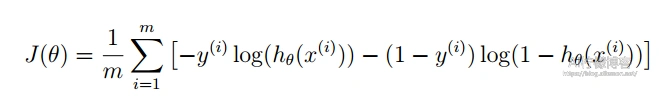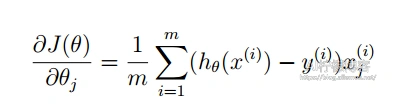θj = θj – α * Grad

 X 0 2 5 -1 -3 4 -0.1 0.5 9 -6 -4 -3 -2 1 8 -7 6 3 1.5 0.5 Y 0 1 1 0 0 1 0 0 1 0 0 0 0 1 1 0 1 1 0 1

fminunc函数：

theta =

-1.7418
1.8137

cost =

0.2007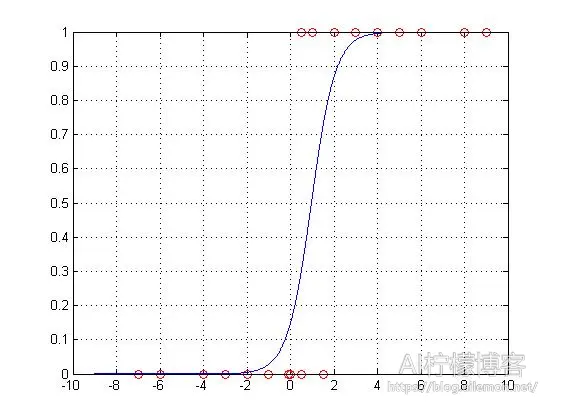theta =

-1.7417
1.8136

cost =

0.2007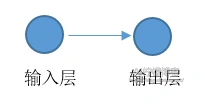《机器学习·周志华》（清华大学出版社）P57 – P60

MATLAB代码：

CostFunction.m

```function [J, grad] = CostFunction(theta, X, y)
%计算对数几率回归的代价函数和梯度
m = length(y); % 训练样例数
J = 0;
J = sum(-y .* log(sigmoid(X * theta)) - (1 - y) .* log(1 - sigmoid(X * theta)) )/m;
grad = (X' * (sigmoid(X * theta) - y))/m;
end```

sigmoid.m

```function g = sigmoid(z)
%计算sigmoid函数值
g = zeros(size(z));
g=1./(1+exp(-1.*z));
end```

1.使用fminunc函数

logistic_fmin.m

```%% 数据
X=[0 2 5 -1 -3 4 -0.1 0.5 9 -6 -4 -3 -2 1 8 -7 6 3 1.5 0.5]';
Y=[0 1 1 0 0 1 0 0 1 0 0 0 0 1 1 0 1 1 0 1]';
plot(X,Y,'ro')
grid on
hold on
%% 初始参数配置
%  获取数据样本数量
[m, n] = size(X);
% 在列向量的左边添加一列全为1的列向量
X = [ones(m, 1) X];
% 初始化拟合参数
initial_theta = zeros(n + 1, 1);
% 计算并显示初始的代价和梯度
[cost, grad] = CostFunction(initial_theta, X, Y)
%% 使用fminunc()函数来最小化代价函数
%  为函数fminunc设置选项
options = optimset('GradObj', 'on', 'MaxIter', 400);
%  运行函数fminunc来获得optimal theta
%  这个函数将返回参数 theta 和代价 cost
[theta, cost] = fminunc(@(t)(CostFunction(t, X, Y)), initial_theta, options)
%% 数据拟合结果展示
x=[ ones(1,1901) ; -9:.01:10]';
y=sigmoid(x * theta);
plot(x(1902:3802),y)```

2.梯度下降自实现

```%% 数据
X=[0 2 5 -1 -3 4 -0.1 0.5 9 -6 -4 -3 -2 1 8 -7 6 3 1.5 0.5]';
Y=[0 1 1 0 0 1 0 0 1 0 0 0 0 1 1 0 1 1 0 1]';
plot(X,Y,'ro')
grid on
hold on
%% 初始参数配置
%  获取数据样本数量
[m, n] = size(X);
% 在列向量的左边添加一列全为1的列向量
X = [ones(m, 1) X];
% 初始化拟合参数
theta=zeros(n + 1, 1);
iterations = 1000; % 设置迭代次数
alpha = 0.5; % 设置学习率
% 计算并显示初始的代价和梯度
[cost, grad] = CostFunction(theta, X, Y)
%% 自制梯度下降来最小化代价函数

J_history = zeros(iterations, 1);
for iter = 1:iterations % 这里不用while的原因是一旦出现发散的情况，程序将陷入死循环
theta = theta - alpha * grad;
end
%% 数据拟合结果展示
x=[ ones(1,1901) ; -9:.01:10]';
y=sigmoid(x * theta);
plot(x(1902:3802),y)
% 显示结果值
theta
J_history(iterations)```

 版权声明本博客的文章除特别说明外均为原创，本人版权所有。欢迎转载，转载请注明作者及来源链接，谢谢。本文地址: https://blog.ailemon.net/2017/02/12/machine-learning-logistic-regression-1/ All articles are under Attribution-NonCommercial-ShareAlike 4.0